# Resistance Measurements

Publish Date: Oct 09, 2019 | 51 Ratings | 2.94 out of 5 | Print | 4 Customer Reviews | Submit your review

## Overview

This digital multimeter measurement tutorial recommends tips and techniques for making accurate resistance measurements with an NI DMM.

### 1. Overview

The NI 4070 Digital Multimeter makes resistance (ohms) measurements using a constant-current technique. A current (amps) is supplied to the resistance (ohms) under test, and the digital multimeter measures the resulting voltage as represented in the figure below: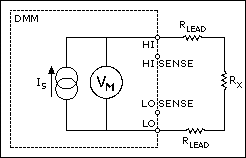With a known current (amps) and voltage, the digital multimeter can determine resistance using Ohm's Law, as follows:

VM = IsRT

where RT is the sum of all resistances, RT = Rx + RLEAD + RLEAD

Therefore, the value of Rx = VM/Is if RLEAD is small or negligible compared to Rx

The NI 4070 Digital Multimeter generates a highly-stable current (amps) source, Is. The table below lists the value of this current (amps) by range:

 Range Current Source Value (nominal) ±5% Is Maximum Test Voltage (Vt) Offset Compensated Ohms Available? 100 Ω 950 µA 105 mV Yes 1 kΩ 950 µA 1.05 V Yes 10 kΩ 95 µA 1.05 V Yes 100 kΩ 9.5 µA 1.05 V No 1 MΩ 9.5 µA 10.5 V No 10 MΩ 0.95 µA 10.5 V No 100 MΩ 0.95 µA *Refer to "Measuring on the 100MOhm Range" section 10.5 V No

Every time you run self-calibration, the current (amps) source recalibrates to an ultra-high stability 10 kΩ reference resistor. While the absolute tolerance of the current (amps) is ±5%, the recalibration process identifies the value to a sub-ppm precision level. The calculated value of resistance (ohms) is corrected to the precision of the ultra-stable reference resistor, assuring accurate measurements.

The NI 4070 Digital Multimeter has two modes of resistance (ohms) measurement: 2-wire and 4-wire . 2-wire and 4-wire apply the same precision current (amps) to the circuit, but the difference between 2-wire and 4-wire resistance (ohms) measurements is how the voltage measurement is made.

### 2. 2-Wire Resistance Measurements

The 2-wire method is commonly used as it is the simplest and most straightforward method. In 2-wire, you can get accurate measurements above 100 kΩ relatively easily. For lower resistance (ohms) values, such as 100 Ω, the interconnecting cabling can add significant resistance (ohms) which can greatly affect your measurement. Copper is the most common and is the recommended cabling. Copper has a temperature coefficient in the 3,000 ppm/ºC range, which can add instability to the measurement. NI recommends Belden 83317 cable. This cable has excellent shielding and insulation resistance (ohms) qualities, and has a resistance of 39 mΩ/ft.

When considering cabling, refer to the following example.

Assume that a test system has 50 feet of copper interconnect cable hooked to a single 100 Ω resistance (ohms), such as a remote sensor device, the cable could easily introduce a resistance (ohms) of ~2 Ω because Belden 83317 has a resistance of 40 mΩ/ft and 50 ft x 40 mΩ/ft = 2 Ω. The temperature coefficient of the resistance (ohms) is as follows:

TC = 2 Ω x 3,000 ppm/ºC = 6 mΩ/ºC

Relative to the 100 Ω resistance (ohms) being measured:

TC = (6 mΩ/ºC)/(100 Ω) = 60 ppm/ºC

The error introduced by the copper not only affects the initial value of resistance (ohms) measured but also introduces a temperature drift into the measurement. The drift with temperature of the copper resistance (60 ppm/ºC in the example above) is much larger than the drift of the resistance (ohms) ranges of the NI 4070 Digital Multimeter (well under 10 ppm/ºC). Recognize, however, that this drift might be perfectly acceptable to the particular measurement being made. Temperature drifts should be considered in the system error budget.

Sometimes the leads can be locally shorted, a measurement made, and then this "offset" and its associated TC subtracted from the subsequent 2-wire resistance (ohms) measurement on the resistors under test. This technique works with careful experimental measurement practice. An outline of the methodology for this technique is as follows, in the context of an automated measurement system, with programmable switching available:

1. Short the leads as close to the resistance (ohms) under test as possible. If the measurement is part of an automated switching system, dedicate a switch channel to a zero value. During the measurement cycle, close the switch to the zero reference. Refer to the following figure: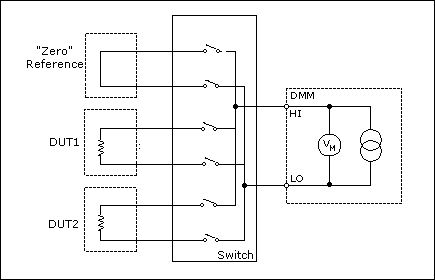• Record the offset of this zero channel.
• Switch to the resistance (ohms) channel you want to use.
• Measure the resistance (ohms) value.
• Subtract the offset value from the resistance (ohms) value on the selected channel. The result is the resistance (ohms) reading you want.

This method is subject to the following caveats:

• If the zero relay has a different contact-resistance (ohms) than the rest of the relays, an error is introduced.
• If possible, you should terminate the zero channel with a cable very close in length to those cables leading to the resistance (ohms) under test, matching the path length as closely as possible.
• This method does not correct for the lead resistance (ohms) of the component you are testing.
• A time penalty occurs in the system and is associated with closing the zero relay and taking the additional measurement.
• The stability of the relay ON-resistance (ohms) may limit this method to a repeatability of about ±20 mΩ.

### 3. 4-Wire Resistance Measurements

For precision measurements with resistances (ohms) below 100 kΩ, 4-wire works more reliably and conveniently than 2-wire. 4-wire requires 4-wire switching and more cabling; however, you may decide the tradeoff is acceptable, depending on the accuracy versus complexity requirements of your system.
The following figure shows a 4-wire resistance (ohms) measurement, including lead and switching resistance: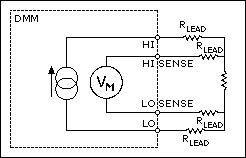Current (amps) is forced through the source leads (HI, LO). As a result, a voltage develops across the resistance (ohms) under test. By measuring the voltage directly across the resistor using the sense leads, the voltage drop of the interconnects (RLEAD) is ignored.

### 4. Offset Compensated Ohms

Offset Compensated Ohms is an NI 4070 Digital Multimeter feature provided for eliminating offset voltages in a resistance (ohms) test system. Whether 2-wire or 4-wire, Offset Compensated Ohms applies and the concept is the same. You have learned about the challenges of removing offset resistances (ohms) from the measurement, however, Offset Compensated Ohms is different—it acts on offset voltages. The measurement involves two cycles as shown in the following figures. The first figure represents the first cycle with the current (amps) source ON: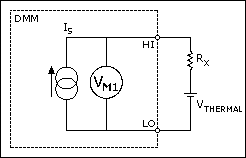The second figure represents the second cycle with the current (amps) source OFF: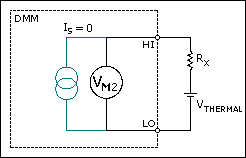The net result is the difference between the two measurements. Since the offset voltage is present in both cycles, it is subtracted out and does not affect the resistance (ohms) calculation.

VOCO = VM1—VM2 = (ISRX + VTHERMAL)—VTHERMAL = ISRX

therefore:

RX = VOCO/IS

When to Use Offset Compensated Ohms
4-wire measurement provides exceptional accuracy if copper interconnects and low-thermal relays are used in the measurement system. However, there are situations when offset voltages present introduce significant errors:

• Switching systems using uncompensated reed relays can have offset voltages >10 µV due to the Kovar lead material used at the device glass seal.
• In-circuit resistance (ohms) measurements, for example, power supply conductors being measured for resistance (ohms), while the circuit is live.
• Measuring the on-resistance (ohms) of batteries, dynamic resistance of forward biased diodes, and so on.

In the case of A above, often a test system is built with switching optimized for other things besides resistance (ohms) measurements. For example, reed relays are common in RF test systems due to their predictable impedance characteristics and high reliability. In such a system you may want to also measure resistances (ohms). It might not be economical to add switching hardware for a few channels of resistance (ohms), and the RF relays may already be present.

In the case of B, an example would be measuring the resistance (ohms) of a power supply bus cable. The resistance (ohms) might be 10 mΩ. If 100 mA is flowing through this resistance, the voltage drop is:

V = 100 mA x 10 mΩ = 1 mV

The digital multimeter without Offset compensation on the 100 Ω range interprets this as 1.05 Ω (recall from the table of Source Current and Maximum Test Voltages that 105 Ω corresponds to nominally 100 mV). With the NI 4070 Digital Multimeter and Offset Compensation, the 1 mV offset is rejected, and the correct value of resistance (ohms) is returned.

In the case of C above, remember that this test only works if the diode is already forward biased. The NI 4070 Digital Multimeter does not supply a DC bias to the diode in Offset Compensated Ohms. The NI 4070 Digital Multimeter does provide the small signal displacement current (amps), but the diode still needs to be biased. The following figure shows this diode example: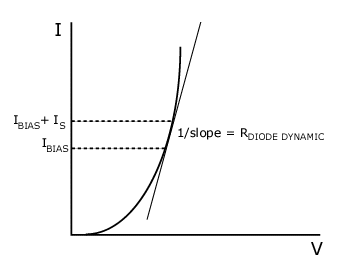To get the dynamic resistance (ohms) of the diode (or transistor) the selection of the appropriate measurement range is key. NI recommends selecting a range with a corresponding current (amps) source value 10% of the bias current (amps) level or less.

NI does not recommend using the digital multimeter to directly measure the series resistance (ohms) of cells greater than 1 V. The NI 4070 Digital Multimeter does not have enough voltage dynamic range to do this on the lower resistance (ohms) ranges. Of course, you can use the digital multimeter in conjunction with an external current (amps) source or load to do this.

As a general rule for measuring resistances (ohms) in the presence of large DC offsets, do not violate the following formula, or the results may be erroneous:

Vos+ IsRx = Vt

where

Vos is the offset voltage (what are trying to compensate out)

Is is the Source Current (dependent on range, refer to the table above)

Rx is the resistance (ohms) being measured (or at least what its upper bound is)

Vt is the Maximum Test Voltage (from table above)

### 5. System Considerations for Resistance Measurements

When measuring resistance (ohms) in a system, it is important to consider the effects of cable resistance. It is also important to notice that interactions between scanning multiple resistances (ohms) and system cabling can present time-dependent problems. These problems can be avoided by following these simple guidelines.

• Remember that as you scan from channel to channel, the current (amps) source must drive the voltage across the resistor to a quiescent level before a meaningful measurement can be made. If there is substantial system capacitance, this capacitance must also be charged by the current (amps) source. If the measurement is started before the capacitance is fully charged, you get an erroneous measurement. For more information, refer to this effect in Settle Time.
• You generally do not encounter much settle time trouble with resistance (ohms) measurements up to 10 kΩ, as long as the system capacitance is <500 pF and you allow a settle time of 2 ms or more.
• Settling time might need to be longer if significant dielectric absorption effects are present in the cables and switching system. Resistance (ohms) values above 100 kΩ are most sensitive to cable capacitance and other dielectric effects. If dielectric absorption is suspect, experimenting by increasing the settle time until the readings no longer change is an empirical way to find the optimum settling.

The following figure illustrates the factors affecting settling time for a resistance (ohms) measurement: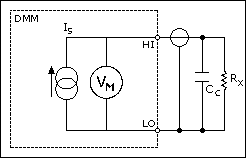Settle Time = kCCRX

where
k = the value related to accuracy required

CC = the capacitance of the cable and switching network

RX = the resistance (ohms) being measuredNote  Remember that if you measure 100 Ω on the 1 MΩ range, the settling time is a function of the measured resistance (ohms) of 100 Ω, not 1 MΩ.

Refer to the Digital Multimeter Measurement Cycle for more information on settle time. Remember that the Offset Compensated Ohms function toggles the current (amps) source on and off. Excessive system capacitance due to cables, in circuit elements, and so on may affect this measurement as it affects it in a scanning system. The Belden 83317 cable described earlier has a capacitance of 35.5 pF/ft and extremely low dielectric absorption.

### 6. Optimizing for High-Resistance Measurements

The techniques required to accurately measure high resistances (ohms) are somewhat different than those required for low resistances. Electrostatic noise pickup becomes a major concern. To prevent problems with noise pickup, shielding must be considered. Refer to "Measuring on the 100 MOhm Range" section for more information.

Shielding
When measuring resistances >100 kΩ proper shielding is critical. High-resistance (ohms) measurements are very sensitive to environmental noise such as power line pick up, changes in electrostatic fields caused by people moving nearby, and even RF pickup. For these reasons, it is strongly encouraged to use shielded cables when measuring high resistances (ohms). For 2-wire measurements the shield should be tied to the LO terminal. For 4-wire measurements, there are two pairs of shielded cables. The first pair should be tied to HI and LO (shield to LO), and the second pair to HI SENSE and LO SENSE (shield to LO SENSE). Refer to the figure below: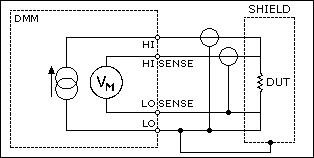### 7. Measuring on the 100 MΩ Range

The 100 MΩ range provides a unique capability, allowing measurements up to 1.05 GΩ, because of the methodology of the measurement and the high–impedance design of the NI 4070 Digital Multimeter. This range allows 2-wire operation only, and Offset Compensated Ohms cannot be enabled.

It is difficult to get a meaningful measurement with resistances (ohms) above 10 MΩ without good shielding—including the resistor under test. Enclose the resistor under test in a metallic box, and connect this enclosure to Input Lo. In the laboratory, even a small box covered with a single, continuous sheet of aluminum foil helps significantly with this kind of measurement.

Noise
The following diagram shows an equivalent circuit for all measurements above the 10 MΩ range.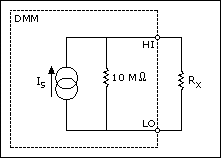The voltage across the resistor is measured by the NI 4070 Digital Multimeter and the resistor is calculated from:

Rx = V /(Is—(V/10 MΩ)

where

Rx = the resistor under test (up to 1.05 GΩ)

V = the voltage measured by the digital multimeter with Rx applied

Is = the source current (nominally 0.95 uA)

For 100 MΩ to 1 GΩ, consider the following:

• The ADC only uses 10% of its range. Typical accuracy values are in the 5% range.
• Any environmental noise or interference is amplified as the resistance (ohms) being measured is increased. The requirement for good shielding increases as the resistor value increases.

The Johnson noise of the resistor is always a factor in the measurement. Calculating from the equation described in the Noise section, the Johnson noise of typical high MΩ resistors is listed below in ppm of reading, assuming a bandwidth of 10 Hz (roughly equivalent to a 100 ms aperture time)

Measurements above 10 MΩ should be made with as long an aperture time as possible, and 100 ms is the minimum recommended value.High-order DC noise rejection  is recommended.

Johnson Noise of Various High -Value Resistors

 Resistor Value R Johnson Noise as ppm of reading (10 Hz bandwidth), p-p1 10 MΩ 1 ppm 30 MΩ 5 ppm 100 MΩ 25 ppm 300 MΩ 140 ppm 1 GΩ 770 ppm

1Represents the "ideal" noise on the best range for that resistance (ohms) measurement due to the Johnson noise of the resistor. Actual noise may be higher, due to environment, poor shielding, and so on.

### 8. Offset Nulling

To perform offset nulling, complete the following steps:

1. Disconnect the DUT from the digital multimeter.
2. Short the input leads to the digital multimeter. In a switching system, you can short the leads with a channel dedicated as a short. Use cables and switches with excellent low thermal voltages and low-path resistance (ohms). Refer to thermal voltages for more information.
3. Record the value of the measurement while the input to the digital multimeter is shorted.
4. Subtract this value from all subsequent measurements.

The subtraction operation can be performed programmatically with your ADE, and the DMM-SFP (Soft Front Panel) has an offset nulling feature.

Customer Reviews
4 Reviews | Submit your review

we need measure a minimum 1 ohms measurement  - Jun 15, 2017

if it s available to measure a value in below 1 ohms measurement in national instrument,send me a feed back

Great Article  - Sep 1, 2010

I find hundreds of technical articles on ni.com that are useful, and don't see NI marketing getting conflicting with technical accuracy either. Both have their place - without successful business, they wouldn't be able to put these types of helpful articles online at all.

Excellent Article  - Jan 9, 2007

Finally a NI article that isn't clogged with company advertisements and recommendations. It gets to the point without bombarding the reader with sales pitches. Would like to see more articles like this. This is the type of articles that made HP the TE greats that they are.

- Mar 5, 2004

You have an excellent page describing resistance measurements. I would add a formula for calculating the resistance in variable lengths and diameters in wires.

### Ratings

Rate this document